#### Categories

1. algebraic geometry
2. algebraic topology
3. analysis of PDEs
4. category theory
5. classical analysis and ODEs
6. combinatorics
7. commutative algebra
8. complex variables
9. differential geometry
10. dynamical systems
11. functional analysis
12. general mathematics
13. general topology
14. geometric topology
15. group theory
16. information theory
17. K-theory and homology
18. logic
19. mathematical physics
20. metric geometry
21. number theory
22. numerical analysis
23. operator algebras
24. optimization and control
25. probability
26. quantum algebra
27. representation theory
28. rings and algebras
29. spectral theory
30. statistics theory
31. symplectic geometry

## Chern connections in the context of CR-geometry

### 1.Preliminaries: Chern theorem

This post is a result of several discussions with Rodiohn Déev.

Fix a complex manifold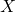and a complex vector bundle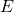over.

Recall that a structure of holomorphic bundle onis given by an operator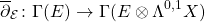which satisfies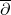-Leibniz identity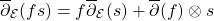and the integrability condition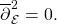For the reader who is more accustomed …

## Demystification of the Willmore integrand

The Willmore energy for a surface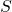in Euclidean 3-space is defined as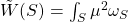, where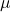is the mean curvature ofand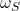its area form. It’s known to be invariant under the conformal transformations (whereas the mean curvature itself is not). White, and later Bryant noticed that the 2-form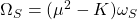, where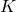stands for the Gaussian curvature, is invariant under conformal transformations; its …

## A shortcut in Kapovich’s proof of Haupt’s theorem

The Teichmüller space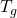of genus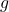curves carries the Hodge bundle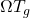, the total space of which maps into the first cohomology space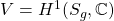via the period map (i. e., a holomorphic 1-form maps into its cohomology class). Haupt’s (or Haupt–Kapovich) theorem describes the image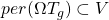in terms of the integral structure on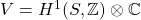and the intersection pairing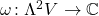. It might be stated as follows.…